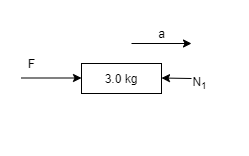# Problem: Blocks with masses of 3.0 kg, 4.0 kg, and 5.0 kg are lined up in a row on a frictionless table. All three are pushed forward by a 20N force applied to the 3.0 kg block.A. How much force does the 4.0 kg block exert on the 5.0 kg block?B. How much force does the 4.0 kg block exert on the 3.0 kg block?

###### FREE Expert Solution

Newton's second law:

$\overline{){\mathbf{\Sigma }}{\mathbf{F}}{\mathbf{=}}{\mathbf{m}}{\mathbf{a}}}$

The mass of the system can be calculated as follows:

M = 3.0 + 4.0 + 5.0 = 12.0 kg

From Newton's second law:

a = F/m = 20/12.0 = 1.67 m/s2

A.

FBD for each block can be drawn as follows:

For the 3.0 kg block, we have81% (40 ratings)###### Problem Details

Blocks with masses of 3.0 kg, 4.0 kg, and 5.0 kg are lined up in a row on a frictionless table. All three are pushed forward by a 20N force applied to the 3.0 kg block.

A. How much force does the 4.0 kg block exert on the 5.0 kg block?

B. How much force does the 4.0 kg block exert on the 3.0 kg block?